#少有人走的路

## C#代码 　实现点在多个二维坐标系中进行换算

（一）坐标系原点旋转的情况

x'=OD+DF=x*cos(20)+y*sin(20)

y'=PC-FC=y*cos(20)-x*sin(20)

```>> 表达式: 300*cos(20)+350*sin(20)
401.614836

>> 表达式: 350*cos(20)-300*sin(20)
226.286374```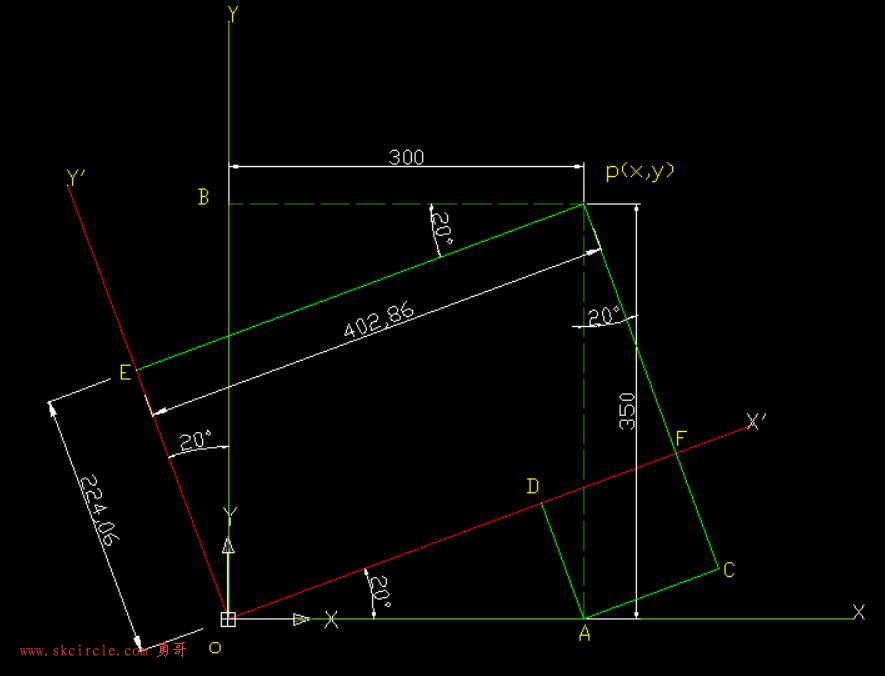（图１）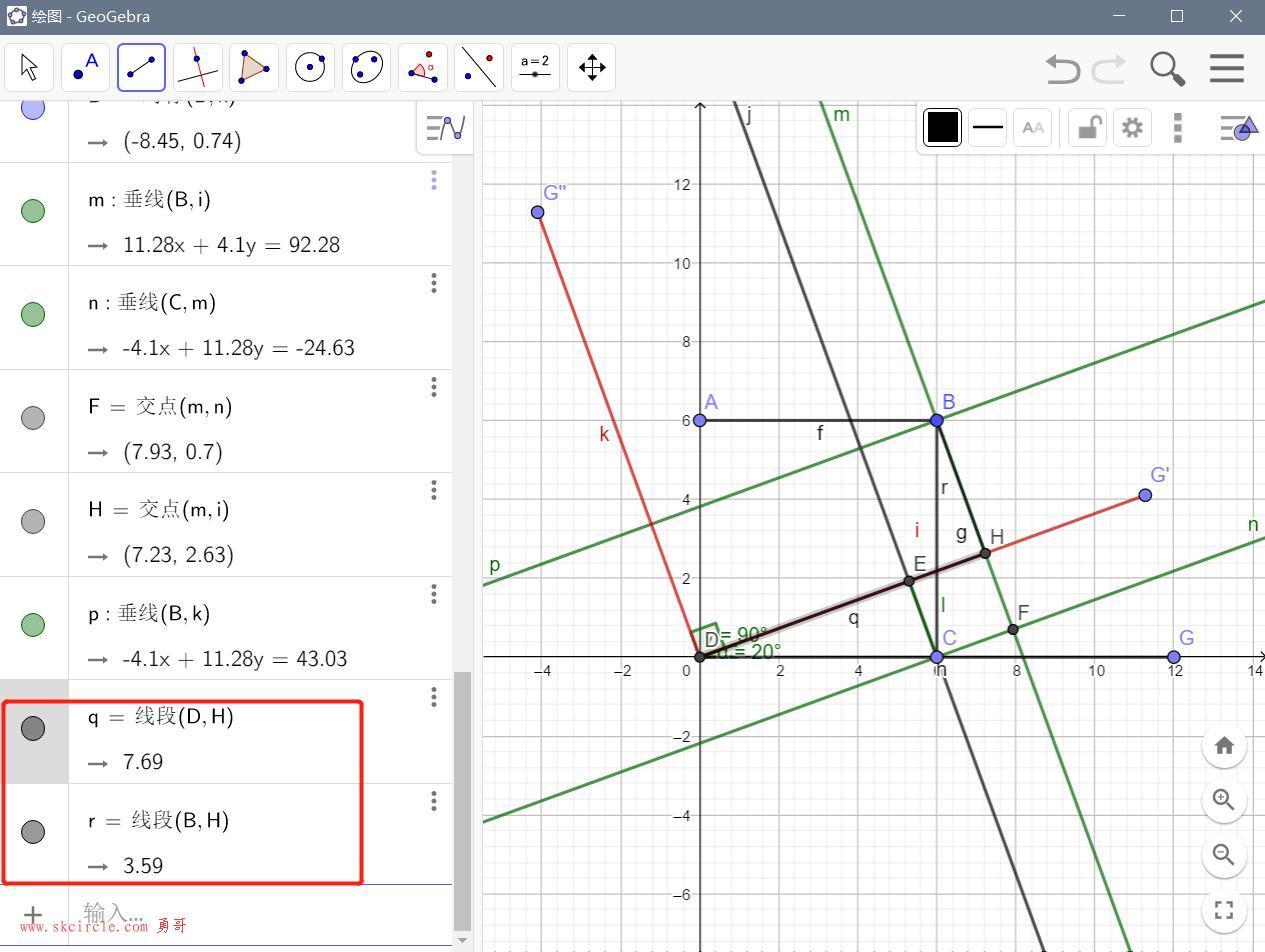（图２）

```命令: cal
>> 表达式: 6*cos(20)+6*sin(20)
7.69027658

>> 表达式: 6*cos(20)-6*sin(20)
3.58603486```

（二）坐标系移动旋转复合变换的情况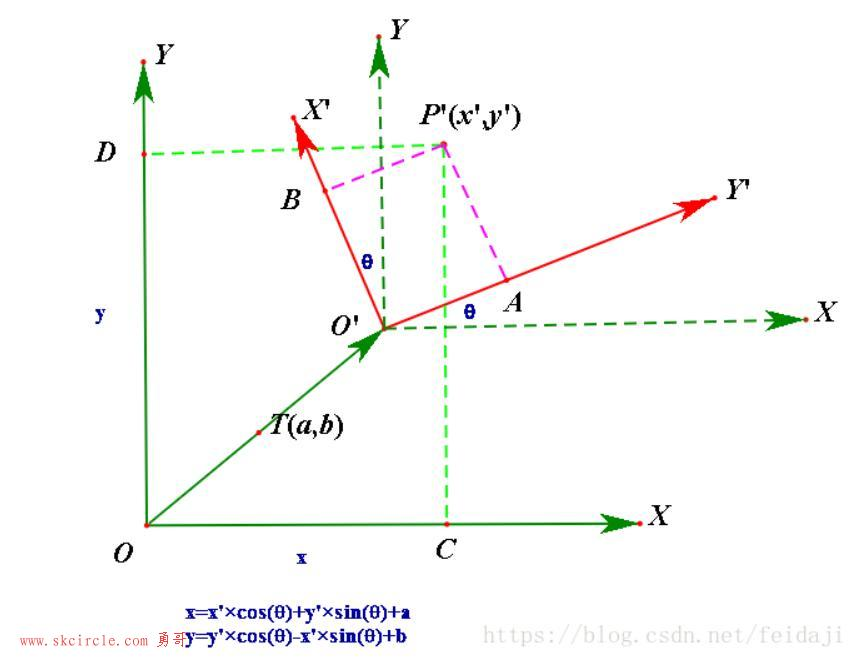（图3）

x=x'*cos(θ)+y'*sin(θ)+a

y=y'*cos(θ)-x'*sin(θ)+b（图4）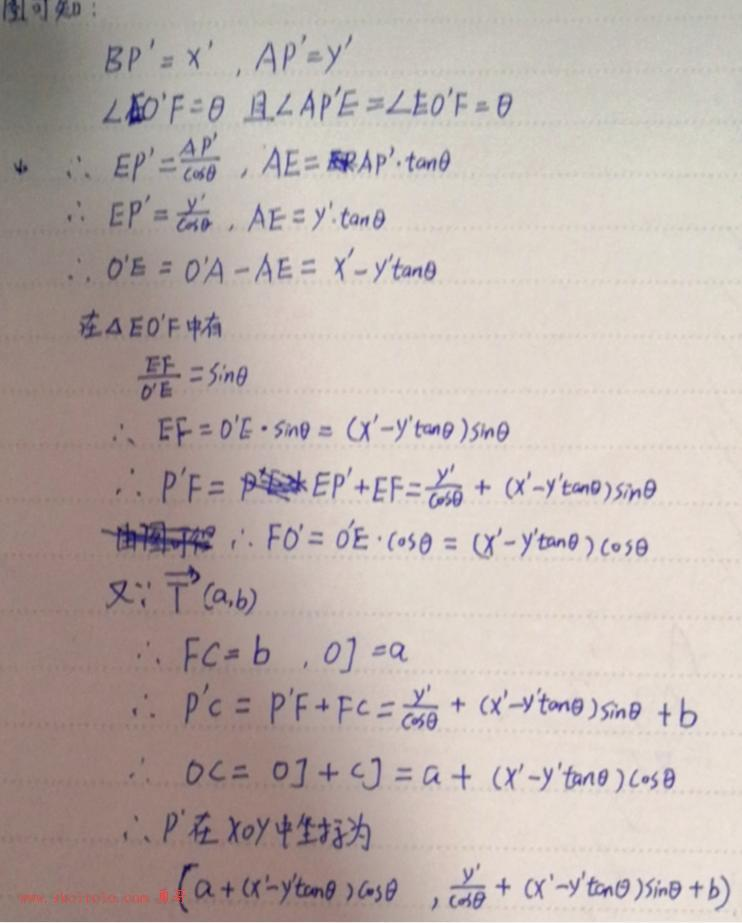（图5）

θ＝20度（图6）

x=x'·cos(θ)+y'·sin(θ)+a
y=y'·cos(θ)-x'·sin(θ)+b

θ=20度

a,b=(4,4)

```命令:CAL
>> 表达式: 2.56*cos(-20)+1.2*sin(-20)+4
5.99518894

>> 表达式: 1.2*cos(-20)-2.56*sin(-20)+4
6.00320271```

（答案是(2.56, 1.2)）

。。。。。

2020/8/15勇哥注：反解推算出来了，见后面的介绍。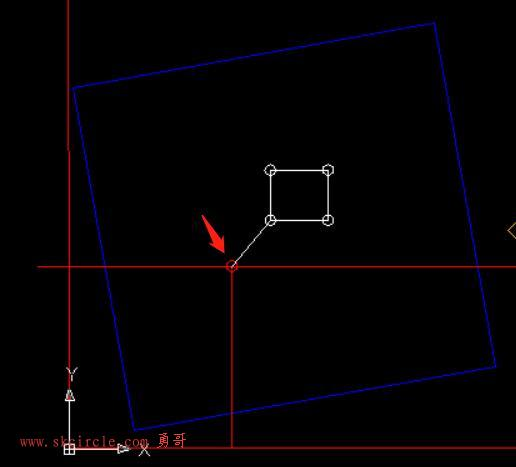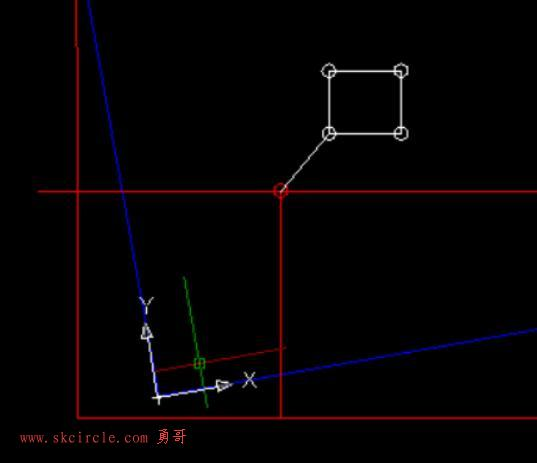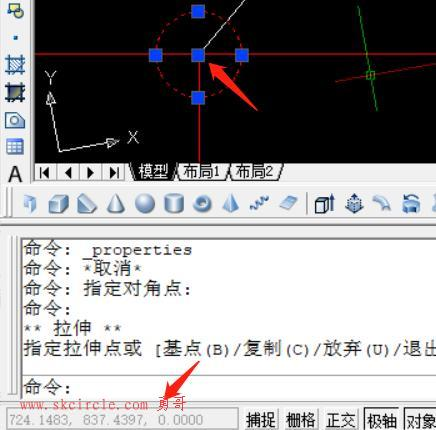```命令: cal
>> 表达式: 724.1483*cos(-10)+837.4397*sin(-10)+369.3
937.026982

>> 表达式: 837.4397*cos(-10)-724.1483*sin(-10)+99.62
1050.08414```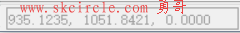（三）反解

（答案是(2.56, 1.2)）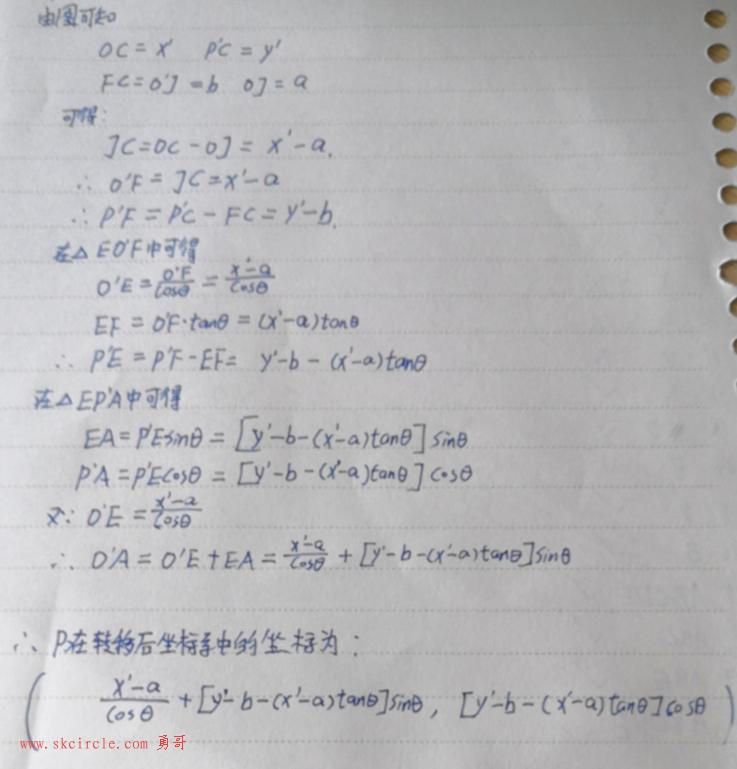---------------------

#转载请注明出处 www.skcircle.com 《少有人走的路》勇哥的工业自动化技术网站。如果需要本贴图片源码等资源，请向勇哥索取。

◎欢迎参与讨论，请在这里发表您的看法、交流您的观点。

«   2020年9月   »
123456
78910111213
14151617181920
21222324252627
282930

查看权限

•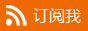• 扫描加本站机器视觉QQ群，验证答案为：halcon• 扫描加站长微信：• 扫描加站长QQ：• 扫描赞赏本站：